# Kähler-Einstein metric

(diff) ← Older revision | Latest revision (diff) | Newer revision → (diff)

A Kähler metric on a complex manifold (or orbifold) whose Ricci tensoris proportional to the metric tensor: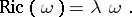This proportionality is an analogue of the Einstein field equation in general relativity. The following conjecture is due to E. Calabi: Letbe a compact connected complex manifold andits first Chern class; then

a) if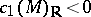, thencarries a unique (Ricci-negative) Kähler–Einstein metricsuch that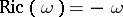;

b) if, then any Kähler class ofadmits a unique (Ricci-flat) Kähler–Einstein metric such that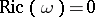.

This conjecture was solved affirmatively by T. Aubin [a1] and S.T. Yau [a8] via studies of complex Monge–Ampère equations, and Kähler–Einstein metrics play a very important role not only in differential geometry but also in algebraic geometry. The affirmative solution of this conjecture gives, for instance, the Bogomolov decomposition for compact Kähler manifolds with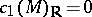. It also implies (see [a2], [a3]):

1) Any Kähler manifold homeomorphic to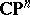is biholomorphic to. Any compact complex surface homotopically equivalent to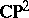is biholomorphic to.

2) In the Miyaoka–Yau inequality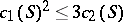, for a compact complex surfaceof general type, equality holds if and only ifis covered by a ball in.

For a Fano manifold(i.e.,is a compact complex manifold with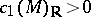), letbe the identity component of the group of all holomorphic automorphisms of. Let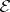be the set of all Kähler–Einstein metricsonsuch that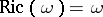. If, thenconsists of a single-orbit (see [a5]). Moreover, the following obstructions to the existence of Kähler–Einstein metrics are known (cf. [a5], [a6]):

Matsushima's obstruction. If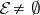, thenis a reductive algebraic group (cf. also Reductive group).

Futaki's obstruction. If, then Futaki's characteris trivial.

Recently (1997), G. Tian [a7] showed some relationship between the existence of Kähler–Einstein metrics onand stability of the manifold, and gave an example of anwith no non-zero holomorphic vector fields satisfying.

The Poincaré metric on the unit open disc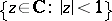(cf. Poincaré model) and the Fubini–Study metric onare both typical examples of Kähler–Einstein metrics. For more examples, see Kähler–Einstein manifold.

For the relationship between Kähler–Einstein metrics and multiplier ideal sheaves, see [a4]. See, for instance, [a2] for moduli spaces of Kähler–Einstein metrics. Finally, Kähler metrics of constant scalar curvature and extremal Kähler metrics are nice generalized concepts of Kähler–Einstein metrics (cf. [a2]).

How to Cite This Entry:
Kähler-Einstein metric. Encyclopedia of Mathematics. URL: http://encyclopediaofmath.org/index.php?title=K%C3%A4hler-Einstein_metric&oldid=11694
This article was adapted from an original article by Toshiki Mabuchi (originator), which appeared in Encyclopedia of Mathematics - ISBN 1402006098. See original article